# Arrays in C Programming

## Arrays Introduction

In order to understand what is array and why array, let us first consider an example. Consider that a course has 7 subjects to be learned. When we program to enter the subject for the course and do some tracking operation on them, how we will code these subjects? With our current knowledge of C, we will create 7 variables of type character to store to store all these subjects. But what is the problem here? All these 7 subjects are of same type and same length – say character and 20 bytes. In addition, if we have to enter the subject names and display them, then we have to write 7 scanf functions to accept the subjects and 7 printf functions to display the subjects. Developer will be tired of typing code right? Imagine, number of subjects are even more or some other situations like enter the number of students in a class / college? How will code look now? It will be lengthier, making code little complex and reducing the readability and interest of developers. How can we keep track of these numbers - number of subjects, number of scanf / printf etc? What if we have to perform some other operations like finding sum / average when marks for these subjects are entered? We can now imagine how complexity of code increases as the number of similar items increases in the code.

If there is any other way of representing such a similar kind of variables which holds any number of similar variable types as well as keeps track of number of items in it, wouldn’t be a great programming? For this reason, C language introduces the concept called Arrays. The array is a datatype or a data structure which contains similar datatypes under one name. It defines a named variable of fixed size which can store similar elements of same datatype and size within it. The datatype of the elements can be of any primitive datatype – int, float, double, char etc. Each element is accessed by using index.

Arrays can be single dimensional or multidimensional array. Single dimensional array stores the data in such way that we have stacked its elements one after the other. When any arrays are created, it allocates contiguous memory locations to its elements. A 2-dimensional array can be imagined as a table with rows and columns. In our example above, we have to store 7 subjects of character type with each of them having size 20. Then we will create an array of type char, with fixed size 7 and each of elements having to store 20 bytes of data. This is an example of 2-Dimensional array.

In C, array indexes start from 0 to max (size)-1. i.e.; if we create an array to store 7 subjects, then array size is 7, which can store its first element at index at 0, second element at index 1, and so on. We will have its last element, 7th element at index 6.

## Array Declaration

Array is a non-primitive datatype. That means, it a variable type which is composed of primitive datatypes. Hence it can be declared as a primitive datatype – by specifying its primitive datatype, array name, size of array. In normal primitive type of variables, we do not specify the size of the variable – which means it can store only one element. But in array when it is declared with its primitive datatype, it calculates the size required for storing one variable of that datatype, and its size tells how many such variables are stored in it. That means, if we declare an array of integers with size 10, then it can store 10 elements of type integer. This is how an array is different from any other primitive datatype, even though we use primitive datatype to declare them.

The general syntax for declaring an array would be as below:

datatype array_name [number of elements / size];

Here datatype may be any primitive datatype, followed by array name. then we add a square bracket to add number of elements that it can hold, followed by end bracket and a semicolon to indicate end of line / declaration.

int intArr ;

This is an array with name intArr of type integer, with 3 elements in it. When an array is being created we have to mention its size while declaring itself. That means size of the array is fixed. We cannot increase it in the middle of the program. In other words an array is a static variable.

An array can be float, double, char etc. it can also be declared in the same way as above.

float flArr ; // array with float type with 10 elements
double dblArr ; // double array with 10 elements
char chrArr ; // character array with 10 elements

Here flArr can store 10 floating type of data within it. Similarly dblArr can store 5 double elements; chrArr can store 7 characters in it.

These are all single dimensional arrays. When they are declared, they get contiguous memory locations assigned to their elements. From below diagram we can understand how a array is created in memory when it is declared. It shows an integer array with N elements. First element of the array occupies 4 bytes of space as the element is of integer type which requires 4 bytes to store the data. Next element in the array gets the memory allocated after 4 bytes i.e.; memory address 10004 and so on. This means contiguous memory is allocated to its elements.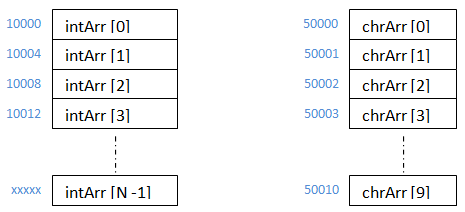Suppose we have created a character array – chrArr. Then each element of it will get one byte of memory allocated to them and every element will be placed one after the other.

## Array Initialization

When we declare an array of any type, it will create memory space for that array to store values. But it will not have any data in it. It will be pointing to the memory addresses assigned to each element of it. Hence if we try to display the values of its elements, it will show some garbage values.

### We can assign values to it elements in different ways :

While declaring the array variable: - Like we initialize any primitive type of variable when we declare them, same method can also be used to initialize arrays. But when we initialize the array, we might have to give values to all of its elements rather than assigning single value. If we assign only one value like any other variable, it will initialize only first element of the array and rest of its element will be automatically initialized to zero in case of numeric datatypes. If the array is character type, it will show nulls to the uninitialized elements of the array.

int intArr  = {10, 20, 30, 40, 50, 60, 70, 80, 90, 100}; // initializes all the 10 elements
int intArr  = {10}; // initializes first element to 10 and rest of them will be zero

``````#include <stdio.h>

void main () {
char intArr  = {‘C’}; // initializes first element to 10 and rest of them will be null

printf ("\nElements of array are:\n");
for (int index = 0; index < 10; index++)
printf ("%c\t", intArr [index]);
}``````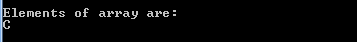``````#include <stdio.h>

void main (){
int intArr  = {10}; // initializes first element to 10 and rest of them will be zero

printf ("\nElements of array are:\n");
for (int index = 0; index < 10; index++)
printf ("%d\t", intArr [index]);
}
``````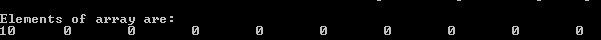If we are initializing the array elements while declaring them, then we need not specify the array size within ‘[]’. It will automatically consider the number of elements as the number of elements that are being initialized. If we need to initialize the elements less than the actual number of elements using this method, then we need to specify the number of elements within’ []’ and can initialize lesser elements.

``````#include <stdio.h>

void main (){
int intArr [] = {10, 20, 30}; // creates 3 elements and initializes them

printf ("\nElements of array are:\n");
for (int index = 0; index < 3; index++)
printf ("%d\t", intArr [index]);
}``````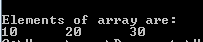## After declaring array variable

We can initialize the array variables after declaring them too. But in this method, we need to explicitly specify the index of the element for which we have to initialize the values. In this method, it initializes only those elements that needs to be initialized and rest of the elements will still have some garbage values. It will not automatically initialize other elements to zero or null.

int intArr ;

intArr  = 10;
intArr  = 20;
intArr  = 30;

``````#include <stdio.h>

void main (){
int intArr ;

intArr  = 10;
intArr  = 20;
intArr  = 30;

printf ("\nElements of array are:\n");
for (int index = 0; index < 10; index++)
printf ("%d\t", intArr [index]);
}``````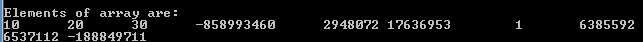## By entering the values from keyboard or input file

We can also initialize the array elements by entering the values using keyboards. User might have requirement to enter his own values each time he executes the program rather than using the same static values like above.

``````#include <stdio.h>

void main (){
int intArr ;

printf ("\nPlease enter 10 array elements :");
for (int index = 0; index < 10; index++)
scanf ("%d", &intArr [index]);

printf ("\nElements of array are:\n");
for (int index = 0; index < 10; index++)
printf ("%d\t", intArr [index]);
}
``````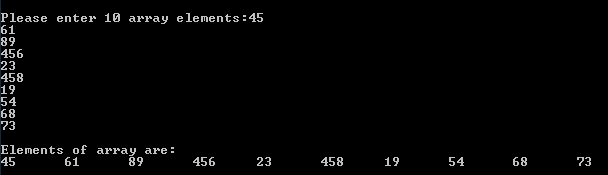## Accessing Array Elements

Arrays can be accessed using their names like any other normal variables. But if we have to access their individual elements, then we have to specify their indexes like 0, 1, 2 etc within ‘[]’.
intArr [0 → indicates first element of the integer array
intArr  → indicates second element of the integer array
intArr  → indicates third element of the integer array
intArr [n-1] → indicates nth element of the integer array

if we need to access all the elements of the array for displaying or entering the values or any other operations, then we can use for loop to increment the indexes and access the elements, rather than specifying each element by specifying the index, like below.

``````#include <stdio.h>

void main(){
int intArr;

printf("\nPlease enter 10 array elements :");
for (int index = 0; index < 10; index++)
scanf("%d", &intArr[index]);

printf("\nElements of array are:\n");
for (int index = 0; index < 10; index++)
printf("intArr[%d] = %d\n", index, intArr[index]);
}
``````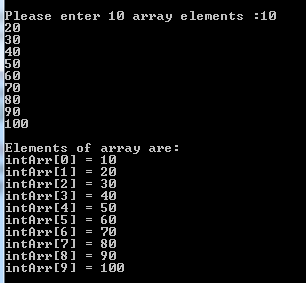Here variable index is used to identify the index of the array intArr and its value is incremented each time in the for loop. Hence it points to each elements of the array from 0 to 9.

Scroll to Top